• DataFrame中set_index()与reset_index()案例详解 set_index() 官方文档 DataFrame.set_index(keys, drop=True, append=False, inplace=False, verify_integrity=False) keys 单个或多个（Multiindex）列名 ...
 DataFrame中set_index()与reset_index()案例详解  set_index() 官方文档
DataFrame.set_index(keys, drop=True, append=False, inplace=False, verify_integrity=False)

keys单个或多个（Multiindex）列名dropdefault True，将作为行索引的列删掉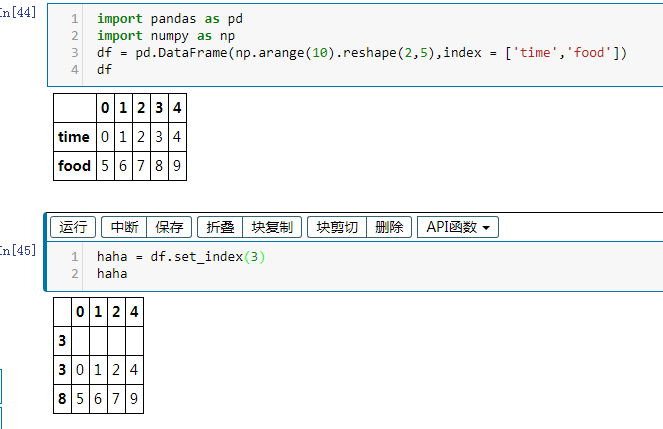reset_index() 官方文档
DataFrame.reset_index(level=None, drop=False, inplace=False, col_level=0, col_fill='')

level将df的原index_label作为新的一列留存，且列名为index，同时自动生成数字indexdropdefalt False，如果是True，删除原来索引inplace下面几个都是Multiindex下的参数，后续研究
常用于在数据清洗过后，对数据重新设置连续行索引。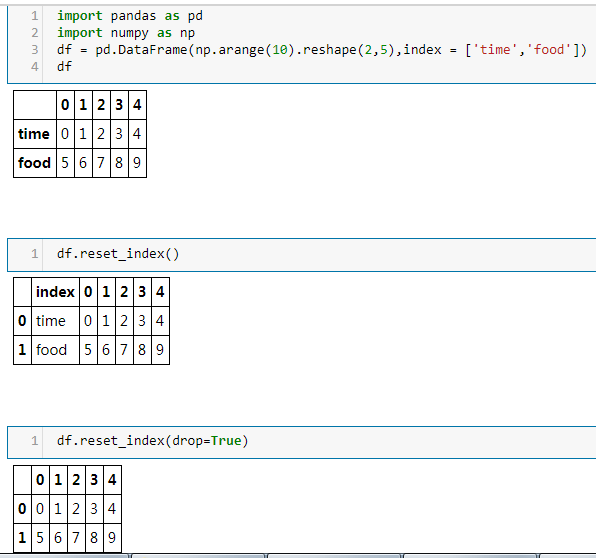展开全文数据清洗
• 文章目录1.pandas.DataFrame.query官方案例2.pandas.DataFrame.reset_index官方案例将reset_index与MultiIndex一起使用 1.pandas.DataFrame.query 官网 DataFrame.query(expr, inplace=False, **kwargs) 描述 使用...


文章目录
1.pandas.DataFrame.query官方案例
2.pandas.DataFrame.reset_index官方案例将reset_index与MultiIndex一起使用

1.pandas.DataFrame.query
官网
DataFrame.query(expr, inplace=False, **kwargs)

描述

使用布尔表达式查询DataFrame的列

参数

expr : str 要计算的查询字符串

inplace : bool 查询是应该修改数据还是返回修改后的副本

**kwargs

返回

DataFrame or None 由提供的查询表达式生成的DataFrame；如果inplace = True，则为None

官方案例
df = pd.DataFrame({'A': range(1, 6),
'B': range(10, 0, -2),
'C C': range(10, 5, -1)})

df
A   B  C C
0  1  10   10
1  2   8    9
2  3   6    8
3  4   4    7
4  5   2    6

df.query('A > B')
A  B  C C
4  5  2    6

# 前面的表达式等价于
df[df.A > df.B]
A  B  C C
4  5  2    6

# 对于名称中有空格的列，可以使用反引号
df.query('B == C C')
A   B  C C
0  1  10   10

# 前面的表达式等价于
df.query('B == C C')
A   B  C C
0  1  10   10

2.pandas.DataFrame.reset_index
官网
DataFrame.reset_index(level=None, drop=False, inplace=False, col_level=0, col_fill='')

描述
重置索引或索引的级别。
重置DataFrame的索引，并使用默认索引。
如果DataFrame具有MultiIndex，则此方法可以删除一个或多个级别

参数

level : int, str, tuple, or list, default None 仅从索引中删除给定的级别,默认情况下删除所有级别。

drop : bool, default False 不要尝试将索引插入dataframe columns,这会将索引重置为默认的整数索引

inplace : bool, default False if True,则在原对象上修改 if False, 则返回修改后的对象

col_level : int or str, default 0 如果列有多个级别，请确定将标签插入到哪个级别。默认情况下，它被插入第一级。

col_fill : object, default ‘’ 如果列具有多个级别，请确定如何命名其他级别。如果为None，则重复索引名称。

返回

DataFrame or None if False,具有新索引的DataFrame；如果inplace = True，则为None

官方案例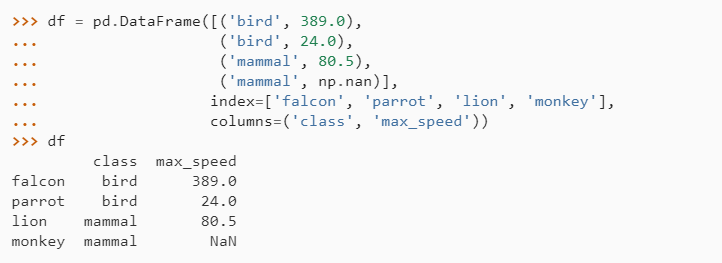重置索引时，会将旧索引添加为列，并使用新的顺序索引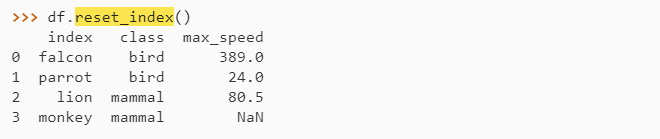我们可以使用drop参数来避免将旧索引添加为列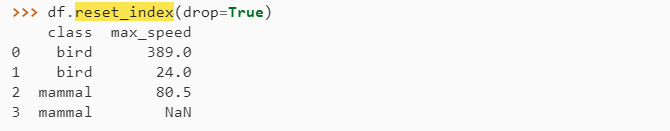将reset_index与MultiIndex一起使用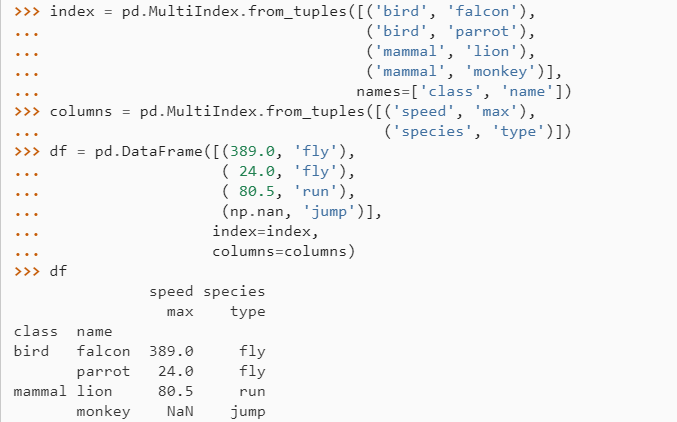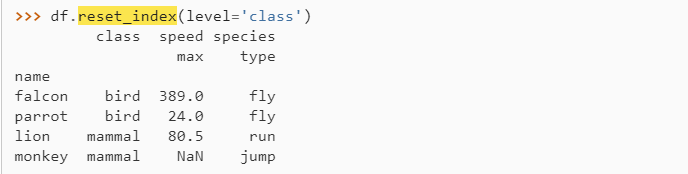如果我们不删除索引，则默认情况下，它位于顶层。 我们可以将其放在另一个级别：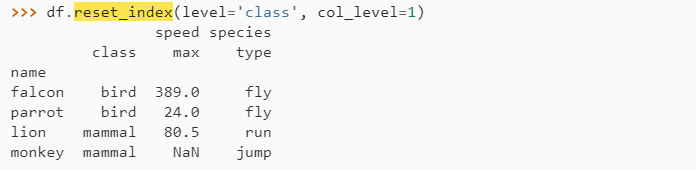当索引插入到另一个级别下时，我们可以使用参数col_fill指定哪个级别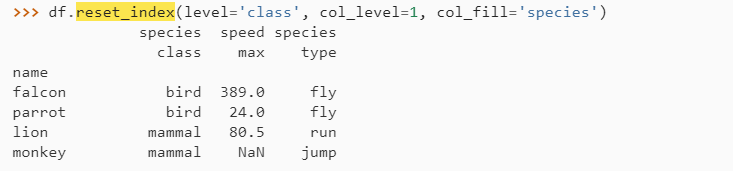如果我们为col_fill指定了不存在的级别，则会创建它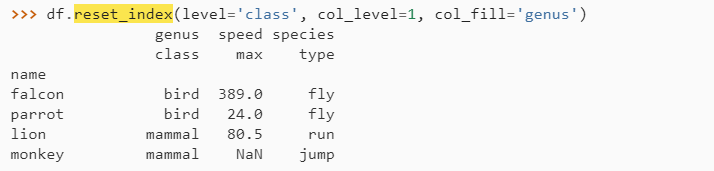展开全文query
• reset_index（）函数作用是重新设置索引，不加drop=True的话，原始的index会作为新的列保存在DataFrame中 reindex()函数作为也是重新设置索引，但不用使用drop=True two_new = two_new.reset_index(drop=True) two...
reset_index（）函数作用是重新设置索引，不加drop=True的话，原始的index会作为新的列保存在DataFrame中 reindex()函数作为也是重新设置索引，但不用使用drop=True
two_new = two_new.reset_index(drop=True)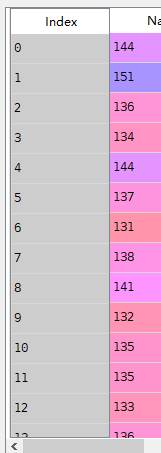two_new = two_new.reset_index()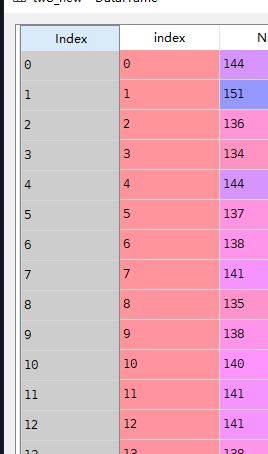two = two.reindex()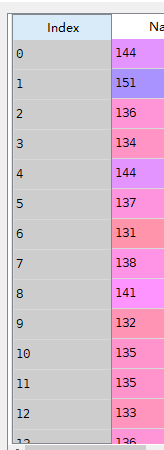展开全文• pandas.DataFramereset_index() 方法 reset_index() 方法将重置DataFrame表的索引，并使用默认索引。 具体是什么意思呢？看以下操作 首先创建一个DF表 该DF表的索引为第一列的值，对该表使用reset_index()...
pandas.DataFrame的 reset_index() 方法
reset_index() 方法将重置DataFrame表的索引，并使用默认索引。
具体是什么意思呢？看以下操作
首先创建一个DF表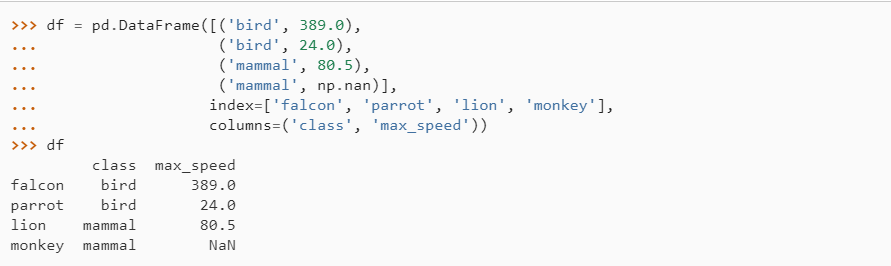该DF表的索引为第一列的值，对该表使用reset_index()方法后，会将旧索引添加为列，并使用新的顺序索引，效果如下：还可使用drop参数直接删除旧索引，不将其添加为该DF表的列：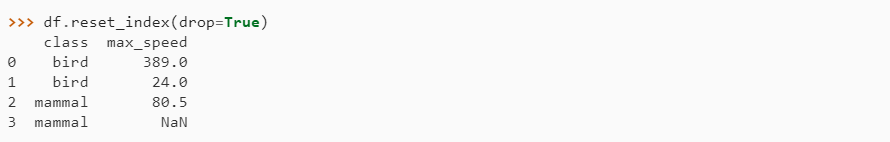展开全文• DataFrame.reset_index(level=None,drop=False,inplace=False,col_level=0,col_fill='')[source] For DataFrame with multi-level index, return new DataFrame with labeling informatio...
• pandas.DataFrame.reset_index() dataframe数据准备 import pandas as pd d={ "cat":[4,"mimi","fish"], "dog":[6,"wangcai","bone"], "monkey":[8,"king","banana"], "tiger":[2,"Simba","meat"] } i=["age",...python pandas
• 问：想问一下，这个在reset_index重新定义索引后，为什么columns不是对应的data吗？ （这本书是《利用python进行数据分析》——学Python的话很经典的一本书） 图片不知道清不清晰，贴下代码 ldata=data.stack()....问答
• reset_index函数功能：示例：In : df Out: 0 1 2 3 4 0 -0.127085 -0.538321 0.641609 -0.020957 0.003503 1 -0.304994 0.157213 0.586962 0.251505 1.022418 2 -0.2...
• DataFrame.reset_index(level=None, drop=False, inplace=False, col_level=0, col_fill='')[source]重置索引或索引的一个级别。重置DataFrame的索引，并使用默认索引。如果DataFrame有一个MultiIndex，此方法可以...
• DataFrame进行切片、融合操作后，DataFrame原有的index不会自动重新排序，这时候我们可以用pandas.DataFrame.reset_index方法让它重新排序： DataFrame.reset_index(level=None, drop=False, inplace=False, ...
• 最近在学习利用python进行数据分析这本书，在数据重塑这一章节中对set_indexreset_index这两个函数的用法有一些不理解，查看了官方文档记录稍微有一些眉目，在这里方便后面查阅。 具体两个函数的效果见代码 import...python 数据分析
• #drop的使用： import pandas as pd df = pd.DataFrame({ 'A': ['A0', 'A1', 'A2', 'A3','A4', 'A5', 'A6', 'A7','A8', 'A9', 'A10', 'A11'], 'B': ['B0', 'B1', 'B2', 'B3','B4', 'B5', 'B6', 'B7...
• Pandas是基于NumPy 的一种工具，该工具是为了解决数据分析任务而创建的。...本文主要介绍一下Pandas中pandas.DataFrame.reset_index方法的使用。 原文地址：Python pandas.DataFrame.reset_index函数方法的使用 ......